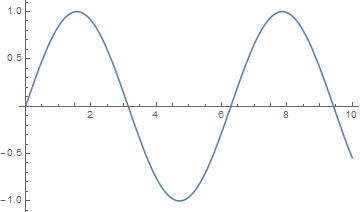# Negative Numbers. HELP (Solved)

So is there any way that I can have an input that goes into the expression block and comes out to be negative?

1 Like

You could have an expression where it’s A*(-1) (Having the number into the A input and the solve input) and it should multiply the number to be a negative, but if the number is already a negative and goes into the expression, you could have a filter that detects if the first number is less than zero, and if it is, then it will skip the expression, since its already a negative.

I don’t know entirely what you need or what situation your in, but this is what I can come up with.

2 Likes

Nope I tried that. It doesn’t work.

1 Like

I don’t understand the question - negative numbers work fine in Expressions.

If you are asking how to negate a number, then you can do this:

`A = A * -1`

3 Likes

Here’s the GAME: Flowlab Game Creator - Game Examples (3)
Go into the “Enlarging Objects” and what I am trying to do is have the object enlarge, but then go back to shrinking.

1 Like

You could have two ease behaviors that lower and enlarge the object instead of using an expression to change the number value.

2 Likes

Do you know how I could do that?

1 Like

I would make an example real quick, but i’m in school so it might take a bit.

1 Like

I would use sin for this, something like:

`Math.sin(A/10) * 40 + 100`

The “10” is the speed it grows/shrinks, the “40” is how much it grows/shrinks, and “100” is the baseline percent.

Here’s an example to drop into your object:

``````
{"data":{"behavior":{"v":"2","nodes":[{"expression":"Math.sin(A/10) * 40  + 100","default0":0,"default1":0,"default2":0,"default3":0,"default4":0,"default5":0,"params":2,"version":2,"tag":"","inputCount":3,"outputCount":1,"name":"Expression","behaviorType":"logic.logic.Expression","x":0,"y":-96,"group":"","id":"eaa60aaaf8fbaa48"},{"inputCount":0,"outputCount":1,"name":"Always","behaviorType":"logic.triggers.Always","x":-360,"y":-96,"group":"","id":"eaa68a6fdb34854a"},{"inputCount":3,"outputCount":1,"name":"Number","behaviorType":"logic.logic.Value","x":-180,"y":-96,"group":"","id":"eaac86bfd37b5847","startVal":0,"roundMode":1},{"inputCount":3,"outputCount":3,"name":"Size","behaviorType":"logic.properties.Scale","x":180,"y":-96,"group":"","id":"eaacf7079e922041"}],"links":[{"input_id":"eaa60aaaf8fbaa48i0","output_id":"eaac86bfd37b5847o0"},{"input_id":"eaa60aaaf8fbaa48i2","output_id":"eaac86bfd37b5847o0"},{"input_id":"eaacf7079e922041i0","output_id":"eaa60aaaf8fbaa48o0"},{"input_id":"eaac86bfd37b5847i2","output_id":"eaa68a6fdb34854ao0"},{"input_id":"eaac86bfd37b5847i2","output_id":"eaa68a6fdb34854ao0"}]}}}
``````
2 Likes

Thank you Grazer! Where do find these equations? I can’t find them online for some reason.

1 Like

I think I just solved this! I actually used another expression block and here’s an image:

2 Likes

That equation I just made up, but using sin() is handy any time you want something to smoothly go back and forth. You feed in an incrementing number, and it returns a value between -1 and 1. Then you can just add/subtract/multiply to scale it how you want4 Likes

I had trouble with this in my early days as wellGlad you figured it out!

1 Like